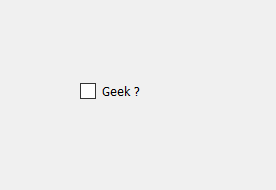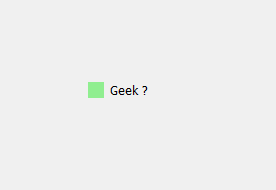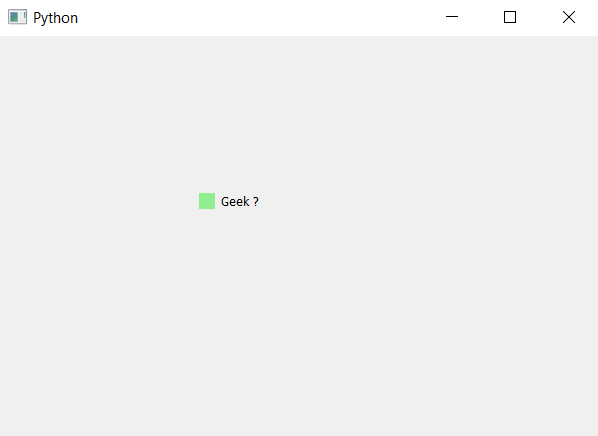# PyQt5 – Change Color of Check Box indicator

• Last Updated : 22 Apr, 2020

Check box basically have two components that are label and a indicator. By default there is no specific color is set to the indicator although we can change the color of the indicator. Below is the representation of normal indicator vs the colored indicator.In order to do this we have to add the background color to the indicator of the check box which can be done using style sheet of indicator below is the style sheet code which can be used with the check box object.

```QCheckBox::indicator
{
background-color : lightgreen;
}
```

Below is the implementation.

 `# importing libraries``from` `PyQt5.QtWidgets ``import` `*` `from` `PyQt5 ``import` `QtCore, QtGui``from` `PyQt5.QtGui ``import` `*` `from` `PyQt5.QtCore ``import` `*` `import` `sys`` ` ` ` `class` `Window(QMainWindow):`` ` `    ``def` `__init__(``self``):``        ``super``().__init__()`` ` `        ``# setting title``        ``self``.setWindowTitle(``"Python "``)`` ` `        ``# setting geometry``        ``self``.setGeometry(``100``, ``100``, ``600``, ``400``)`` ` `        ``# calling method``        ``self``.UiComponents()`` ` `        ``# showing all the widgets``        ``self``.show()`` ` `    ``# method for widgets``    ``def` `UiComponents(``self``):`` ` `        ``# creating the check-box``        ``checkbox ``=` `QCheckBox(``'Geek ?'``, ``self``)`` ` `        ``# setting geometry of check box``        ``checkbox.setGeometry(``200``, ``150``, ``100``, ``30``)`` ` `        ``# adding background color to indicator``        ``checkbox.setStyleSheet(``"QCheckBox::indicator"``                               ``"{"``                               ``"background-color : lightgreen;"``                               ``"}"``)`` ` ` ` ` ` `# create pyqt5 app``App ``=` `QApplication(sys.argv)`` ` `# create the instance of our Window``window ``=` `Window()`` ` `# start the app``sys.exit(App.``exec``())`

Output :My Personal Notes arrow_drop_up